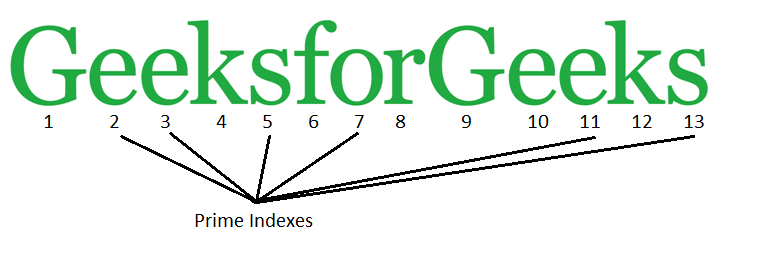# Program to print characters present at prime indexes in a given string

Given a string, our task is to print the characters present at prime index.

Examples :

```Input : I love programming
Output : lv gan
Explanation :
prime index characters in a string are : lv gan

Input : Happy coding everyone
Output : apycn ro
```## Recommended: Please try your approach on {IDE} first, before moving on to the solution.

Approach :

1. Use two loops to divide the numbers upto the value of length of string.
2. Increment variable result when the remainder is 0.
3. If the variable result = 1, then print the corresponding character.

Below is the implementation of above approach :

## C++

 `// C++ Program to print Characters at ` `// Prime index in a given String ` `#include ` `using` `namespace` `std; ` ` `  `bool` `isPrime(``int` `n) ` `{ ` `    ``// Corner case ` `    ``if` `(n <= 1) ``return` `false``; ` ` `  `    ``// Check from 2 to n-1 ` `    ``for` `(``int` `i = 2; i < n; i++) ` `        ``if` `(n % i == 0) ` `            ``return` `false``; ` ` `  `    ``return` `true``; ` `} ` ` `  `// Function to print  ` `// character at prime index ` `void` `prime_index(string input) ` `{ ` `    ``int` `n = input.length(); ` ` `  `    ``// Loop to check if ` `    ``// index prime or not ` `    ``for` `(``int` `i = 2; i <= n; i++)  ` `        ``if` `(isPrime(i))  ` `            ``cout << input[i - 1];          ` `} ` ` `  `// Driver Code ` `int` `main() ` `{ ` `    ``string input = ``"GeeksforGeeks"``; ` `    ``prime_index(input); ` `    ``return` `0; ` `} `

## Java

 `// Java Program to print  ` `// Characters at Prime index  ` `// in a given String ` `class` `GFG ` `{ ` `    ``static` `boolean` `isPrime(``int` `n) ` `    ``{ ` `        ``// Corner case ` `        ``if` `(n <= ``1``) ``return` `false``; ` `     `  `        ``// Check from 2 to n-1 ` `        ``for` `(``int` `i = ``2``; i < n; i++) ` `            ``if` `(n % i == ``0``) ` `                ``return` `false``; ` `     `  `        ``return` `true``; ` `    ``} ` `     `  `    ``// Function to print  ` `    ``// character at prime index ` `    ``static` `void` `prime_index(String input) ` `    ``{ ` `        ``int` `n = input.length(); ` `     `  `        ``// Loop to check if  ` `        ``// index prime or not ` `        ``for` `(``int` `i = ``2``; i <= n; i++)  ` `            ``if` `(isPrime(i))  ` ` `  `                ``System.out.print ` `                ``(input.charAt(i - ``1``));          ` `    ``} ` `     `  `    ``// Driver code  ` `    ``public` `static` `void` `main (String[] args) ` `    ``{ ` `        ``String input = ``"GeeksforGeeks"``; ` `         `  `        ``prime_index(input); ` `    ``} ` `} ` ` `  `// This code is contributed by Anant Agarwal. `

## Python

 `# Python3 program to print ` `# Characters at Prime index  ` `# in a given String ` ` `  `def` `isPrime(n): ` ` `  `    ``# Corner case ` `    ``if` `n <``=` `1``: ` `        ``return` `False` ` `  `    ``# Check from 2 to n-1 ` `    ``for` `i ``in` `range``(``2``, n): ` `        ``if` `n ``%` `i ``=``=` `0``: ` `            ``return` `False``; ` ` `  `    ``return` `True` ` `  `# Function to print ` `# character at prime index ` `def` `prime_index (``input``): ` `    ``p ``=` `list``(``input``) ` `    ``s ``=` `"" ` `     `  `    ``# Loop to check if ` `    ``# index prime or not ` `    ``for` `i ``in` `range` `(``2``, ``len``(p) ``+` `1``): ` `        ``if` `isPrime(i): ` `            ``s ``=` `s ``+` `input``[i``-``1``] ` `    ``print` `(s) ` `         `  `# Driver Code ` `input` `=` `"GeeksforGeeks"` `prime_index(``input``)  `

## C#

 `// C# Program to print Characters  ` `// at Prime index in a given String ` `using` `System; ` ` `  `class` `GFG ` `{ ` `    ``static` `bool` `isPrime(``int` `n) ` `    ``{ ` `        ``// Corner case ` `        ``if` `(n <= 1) ``return` `false``; ` `     `  `        ``// Check from 2 to n-1 ` `        ``for` `(``int` `i = 2; i < n; i++) ` `            ``if` `(n % i == 0) ` `                ``return` `false``; ` `     `  `        ``return` `true``; ` `    ``} ` `     `  `    ``// Function to print character  ` `    ``// at prime index ` `    ``static` `void` `prime_index(``string` `input) ` `    ``{ ` `        ``int` `n = input.Length; ` `     `  `        ``// Loop to check if  ` `        ``// index prime or not ` `        ``for` `(``int` `i = 2; i <= n; i++)  ` `            ``if` `(isPrime(i))  ` ` `  `                 `  `                ``Console.Write(input[i - 1]);          ` `    ``} ` `     `  `    ``// Driver code  ` `    ``public` `static` `void` `Main () ` `    ``{ ` `        ``string` `input = ``"GeeksforGeeks"``; ` `         `  `        ``prime_index(input); ` `    ``} ` `} ` ` `  `// This code is contributed by Vt_m. `

## PHP

 ` `

Output :

```eesoes
```

Optimizations :
For large strings, we can use Sieve of Eratosthenes to efficiently find all prime numbers smaller than or equal to length of string.

My Personal Notes arrow_drop_upCheck out this Author's contributed articles.

If you like GeeksforGeeks and would like to contribute, you can also write an article using contribute.geeksforgeeks.org or mail your article to contribute@geeksforgeeks.org. See your article appearing on the GeeksforGeeks main page and help other Geeks.

Please Improve this article if you find anything incorrect by clicking on the "Improve Article" button below.

Improved By : jit_t

Article Tags :
Practice Tags :

Be the First to upvote.

Please write to us at contribute@geeksforgeeks.org to report any issue with the above content.